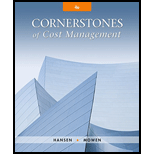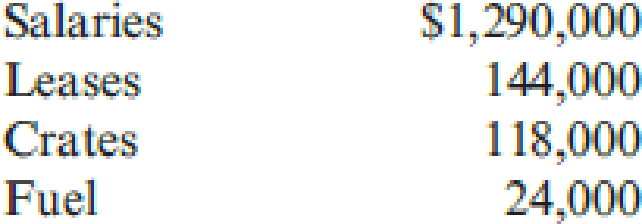# Novo, Inc., wants to develop an activity flexible budget for the activity of moving materials. Novo uses eight forklifts to move materials from receiving to stores. The forklifts are also used to move materials from stores to the production area. The forklifts are obtained through an operating lease that costs $18,000 per year per forklift. Novo employs 25 forklift operators who receive an average salary of$50,000 per year, including benefits. Each move requires the use of a crate. The crates are used to store the parts and are emptied only when used in production. Crates are disposed of after one cycle (two moves), where a cycle is defined as a move from receiving to stores to production. Each crate costs $1.80. Fuel for a forklift costs$3.60 per gallon. A gallon of gas is used every 20 moves. Forklifts can make three moves per hour and are available for 280 days per year, 24 hours per day (the remaining time is downtime for various reasons). Each operator works 40 hours per week and 50 weeks per year. Required: 1. Prepare a flexible budget for the activity of moving materials, using the number of cycles as the activity driver. 2. Calculate the activity capacity for moving materials. Suppose Novo works at 80 percent of activity capacity and incurs the following costs: Prepare the budget for the 80 percent level and then prepare a performance report for the moving materials activity. 3. Calculate and interpret the volume variance for moving materials. 4. Suppose that a redesign of the plant layout reduces the demand for moving materials to one-third of the original capacity. What would be the budget formula for this new activity level? What is the budgeted cost for this new activity level? Has activity performance improved? How does this activity performance evaluation differ from that described in Requirement 2? Explain.### Cornerstones of Cost Management (C...

4th Edition
Don R. Hansen + 1 other
Publisher: Cengage Learning
ISBN: 9781305970663

#### Solutions

Chapter
Section### Cornerstones of Cost Management (C...

4th Edition
Don R. Hansen + 1 other
Publisher: Cengage Learning
ISBN: 9781305970663
Chapter 12, Problem 25P
Textbook Problem
168 views

## Novo, Inc., wants to develop an activity flexible budget for the activity of moving materials. Novo uses eight forklifts to move materials from receiving to stores. The forklifts are also used to move materials from stores to the production area. The forklifts are obtained through an operating lease that costs $18,000 per year per forklift. Novo employs 25 forklift operators who receive an average salary of$50,000 per year, including benefits. Each move requires the use of a crate. The crates are used to store the parts and are emptied only when used in production. Crates are disposed of after one cycle (two moves), where a cycle is defined as a move from receiving to stores to production. Each crate costs $1.80. Fuel for a forklift costs$3.60 per gallon. A gallon of gas is used every 20 moves. Forklifts can make three moves per hour and are available for 280 days per year, 24 hours per day (the remaining time is downtime for various reasons). Each operator works 40 hours per week and 50 weeks per year.Required: 1. Prepare a flexible budget for the activity of moving materials, using the number of cycles as the activity driver. 2. Calculate the activity capacity for moving materials. Suppose Novo works at 80 percent of activity capacity and incurs the following costs:Prepare the budget for the 80 percent level and then prepare a performance report for the moving materials activity. 3. Calculate and interpret the volume variance for moving materials. 4. Suppose that a redesign of the plant layout reduces the demand for moving materials to one-third of the original capacity. What would be the budget formula for this new activity level? What is the budgeted cost for this new activity level? Has activity performance improved? How does this activity performance evaluation differ from that described in Requirement 2? Explain.

1.

To determine

Construct a flexible budget for N Company for the activity of moving materials using number of cycles as the activity driver.

### Explanation of Solution

Flexible budget: A flexible budget is a statement of estimate regarding revenues and expenses for a future period that can be modified depending on the level of output.

 Resource Fixed ($) Variable Salaries 1,250,000 (1) - Lease 144,000 (2) - Crates -$1.80 Fuel - (3) $0.36 Total 1,394,000$2.16

Table (1)

Working notes:

(1) Calculate the total salary expenses.

Salaries=Number of forklift operators×Average salary per year=25×$50,000=1,250,000 (2) Calculate the total lease payable 2. To determine Compute the activity capacity of N Company for moving materials assuming that N Company works at 80 percentage activity capacity. Construct a budget for 80 percent capacity level and also prepare a performance report for the moving materials activity if N Company incurred the following cost. Salaries -$1,290,000

Lease - $144,000 Crates -$118,000

Fuel - \$24,000

3.

To determine

Compute the volume variance of N Company and interpret the results.

4.

To determine

Compute the budget formula and new activity level if a redesign of the plant layout reduces the demand for moving materials to one-third of the original capacity. Provide information and evaluate the calculated result with proper explanation.

### Still sussing out bartleby?

Check out a sample textbook solution.

See a sample solution

#### The Solution to Your Study Problems

Bartleby provides explanations to thousands of textbook problems written by our experts, many with advanced degrees!

Get Started

Find more solutions based on key concepts
BETA COEFFICIENT Given the following information determine the beta coefficient for Stock J that is consistent ...

Fundamentals of Financial Management, Concise Edition (with Thomson ONE - Business School Edition, 1 term (6 months) Printed Access Card) (MindTap Course List)

What is the variable cost ratio? The contribution margin ratio? How are the two ratios related?

Managerial Accounting: The Cornerstone of Business Decision-Making

What are the major elements of design planning?

Foundations of Business (MindTap Course List)